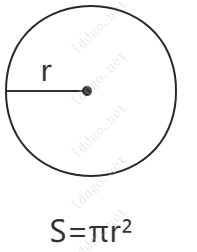Online Tools

# Perimeter Calculator Online

TAG math-tool

The number of center angles,unit °
arc length
semi-major axis
semi-minor axis
triangle side length
triangle side length
triangle side length
length
length
width
side a
side b
side a
side b
side c
side d
side a
side b
side c
side dRelated Tools
Perimeter Calculator Online-summary

The online perimeter calculator supports calculating the perimeter of shapes such as circles, sectors, ellipses, triangles, squares, rectangles, and quadrilaterals, and displays the perimeter calculation formulas and schematic diagrams for corresponding shapes.

Perimeter Calculator Online-instructions

Online perimeter calculator, calculates the perimeter of various shapes and displays the calculation formula for the corresponding shape perimeter.

1. Shape : Choose to calculate the perimeter of what shape. This tool supports calculating the perimeter of shapes such as circles, sectors, ellipses, triangles, squares, rectangles, parallelograms, quadrilaterals, trapezoids, etc, and display the schematic diagram and formula for calculating the perimeter of the corresponding shape.
2. After selecting the corresponding shape, please enter the corresponding parameters as required. Click Calculate to obtain the corresponding perimeter result. Please pay attention to entering the parameters correctly according to the parameter prompts.
3. The calculated perimeter does not have a specific unit, and the unit of perimeter depends on the input parameter's unit.
4. Formula for calculating the perimeter of each shape:
1. Circle : , where r is the radius and π is the pi.
2. Fan-shaped ( angle ) : , where n is the angle of the Central angle, r is the radius, and π is the pi.
3. Fan-shaped ( arc length ) : , where r is the radius and I is the arc length.
4. Ellipse : , where π is pi, a is the length of the semi major axis, b is the length of the semi minor axis. The perimeter of an ellipse is approximated using Ramanujan's formula.
5. Triangle : , where a, b, and c are the length of the three sides of a triangle.
6. Square : , where a is the side length.
7. Rectangle : , where a and b are the length and width of the rectangle.
8. Parallelogram : , where a and b are the side lengths of parallelograms.
9. Quadrilateral : , where a, b, c, and d are the side lengths of the quadrilateral.
10. Trapezium : , where a, b, c, and d are the side lengths of the trapezoid.